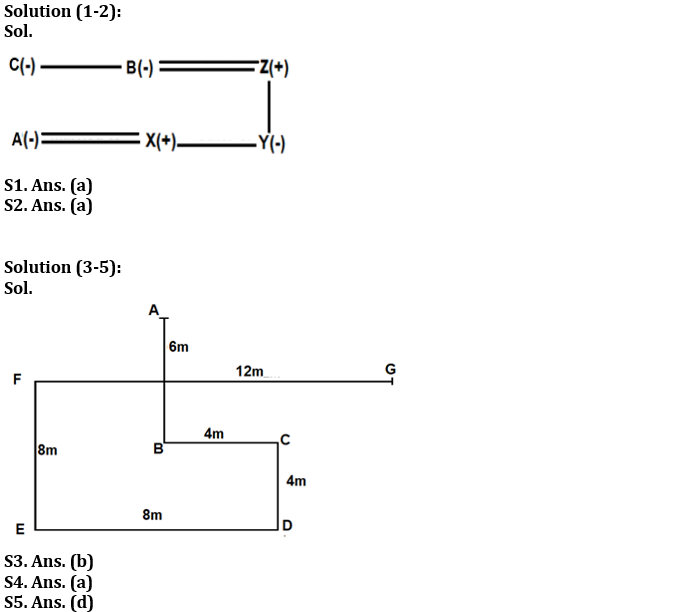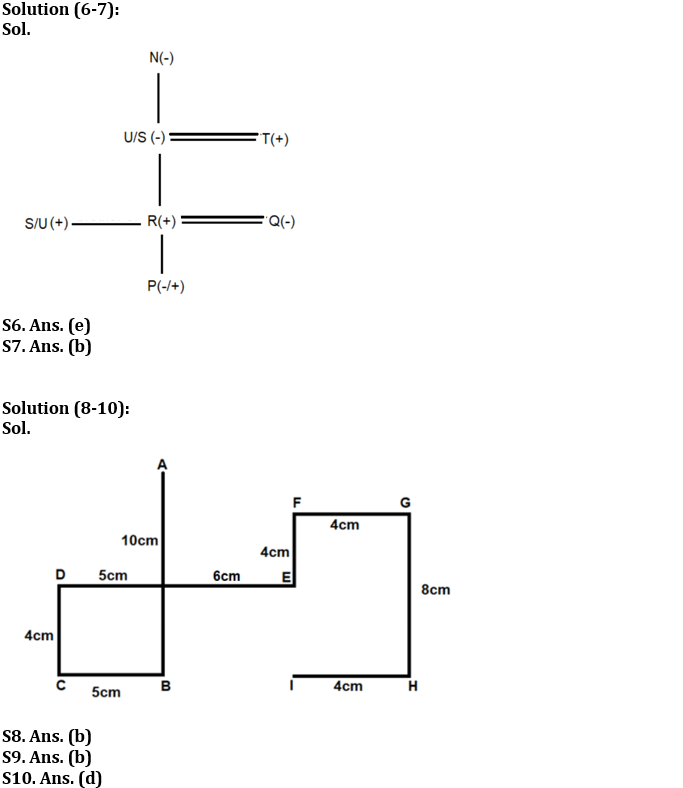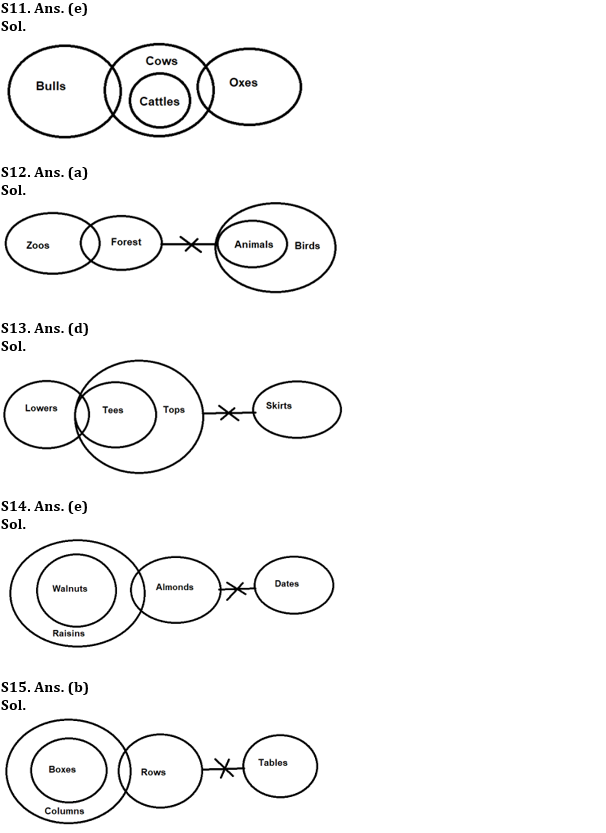Latest Banking jobs   »

# Reasoning Ability Quiz For IBPS RRB PO Prelims 2022- 30th June

Directions (1-2): Answer the questions based on the information given below.
A family consists of six members A, B, X, Z, C and Y. There is no single parent in the family. There are only two generations in the family. Y is the only daughter of Z. B is the mother of X. C is the maternal aunt of X. A is the daughter-in-law of Z.

Q1. How is X related to Z?
(a) Son
(b) Daughter
(c) Brother
(d) Sister
(e) Can’t be determined

Q2. How will be the husband of B related to C?
(a) Brother-in-law
(b) Son-in-law
(c) Father-in-law
(d) Father
(e) None of these

Directions (3-5): Answer the questions based on the information given below.
A person starts walking in south direction from point A. After walking 6m, he reached at point B. From point B, he takes a left turn and walks for 4m to reach point C. Then, he turns right and walks for 4m towards D. Now, he again turns right from point D and walks for 8m to reach point E. He turns right from point E and walks for 8m till point F. Finally, he again turns right from point F and walks for 12m till point G.

Q3. In which direction is Point A with respect to Point G?
(a) North-East
(b) North-west
(c) North
(d) West
(e) None of these

Q4. What is the shortest distance between point C and point G?
(a) √32m
(b) √38m
(c) √36m
(d) √40m
(e) None of these

Q5. In which direction is Point E with respect to Point A?
(a) North-East
(b) North-west
(c) North
(d) South-west
(e) None of these

Directions (6-7): Answer the questions based on the information given below.
There are seven members in a family of four generations. T is father of R’s brother. Q is mother of P. N is mother-in-law of T. R is married. The genders of S and U are opposite to each other. Q is not married to T.

Q6. How is S related to Q?
(a) Father-in-law
(b) Brother-in-law
(c) Son-in-law
(d) Mother-in-law
(e) Can’t be determined

Q7. How is R related to N?
(a) Grandfather
(b) Grandson
(c) Sister
(d) Mother
(e) None of these

Directions (8-10): Answer the questions based on the information given below.
There are nine points A, B, C, D, E, F, G, H and I, drawn on a sheet of paper. B is 10cm to the south of A. D is 11cm to the west of E. D is 4cm to the north of C. G is 4cm to the east of F. H is 8cm to the south of G.I is 4cm to the west of H. C is 5cm to the west of B. F is 4cm to the north of E.

Q8. What is the direction of point A with respect to point I?
(a) North-East
(b) North-west
(c) North
(d) South-west
(e) None of these

Q9. What is the shortest distance between point C and point H?
(a) 13m
(b) 15m
(c) 12m
(d) 18m
(e) None of these

Q10. What is the direction of point C with respect to point G?
(a) North-East
(b) North-west
(c) South-east
(d) South-west
(e) None of these

Directions (11-15): In the question below, three statements are given followed by the conclusions. You have to take the given statements to be true even if they seem to be at variance with commonly known facts. Read all the conclusions and then decide which of the given conclusions logically follows from the given statements disregarding commonly known facts.

Q11. Statements: Only a few Bulls are Cows. Some Oxes are Cows. Only Cows are Cattles.
Conclusions:
I. Some Bulls are Cattles.
II. All Cows can be Bulls.
(a) If only conclusion I follows
(b) If only conclusion II follows
(c) If either conclusion I or conclusion II follows
(d) If both conclusions I and II follow
(e) If neither conclusion I nor conclusion II follows

Q12. Statements: Only a few Zoos are Forests. No Forests are Animals. All Animals are Birds.
Conclusions:
I. Some Zoos are not Forests.
II. Some Animals are not Zoo.
(a) If only conclusion I follows
(b) If only conclusion II follows
(c) If either conclusion I or conclusion II follows
(d) If both conclusions I and II follow
(e) If neither conclusion I nor conclusion II follows

Q13. Statements: Some Lowers are Tees. All Tees are Tops. No Tops are Skirts.
Conclusions:
I. Some Lowers are not Skirts
II. No Skirts are Tees
(a) If only conclusion I follows
(b) If only conclusion II follows
(c) If either conclusion I or conclusion II follows
(d) If both conclusions I and II follow
(e) If neither conclusion I nor conclusion II follows

Q14. Statements: All Walnuts are Raisins. Some Raisins are Almonds. No Almonds are Dates.
Conclusions:
I. Some Raisins are not Almonds.
II. Some Walnuts are Dates.
(a) If only conclusion I follows
(b) If only conclusion II follows
(c) If either conclusion I or conclusion II follows
(d) If both conclusions I and II follow
(e) If neither conclusion I nor conclusion II follows

Q15. Statements: All Boxes are Columns. Some Columns are Rows. No Rows are Tables.
Conclusions:
I. No Boxes are Tables
II. Some Columns are not Tables
(a) If only conclusion I follows
(b) If only conclusion II follows
(c) If either conclusion I or conclusion II follows
(d) If both conclusions I and II follow
(e) If neither conclusion I nor conclusion II follows

Solutions#### Congratulations!Incorrect details? Fill the form again here

•Quantitative Aptitude Quiz For IBPS RRB ...
•Reasoning Ability Quiz For IBPS RRB PO C...
•Reasoning Ability Quiz For IBPS RRB PO C...
•Quantitative Aptitude Quiz For IBPS RRB ...
•Reasoning Ability Quiz For IBPS RRB PO C...
•Quantitative Aptitude Quiz For IBPS RRB ...